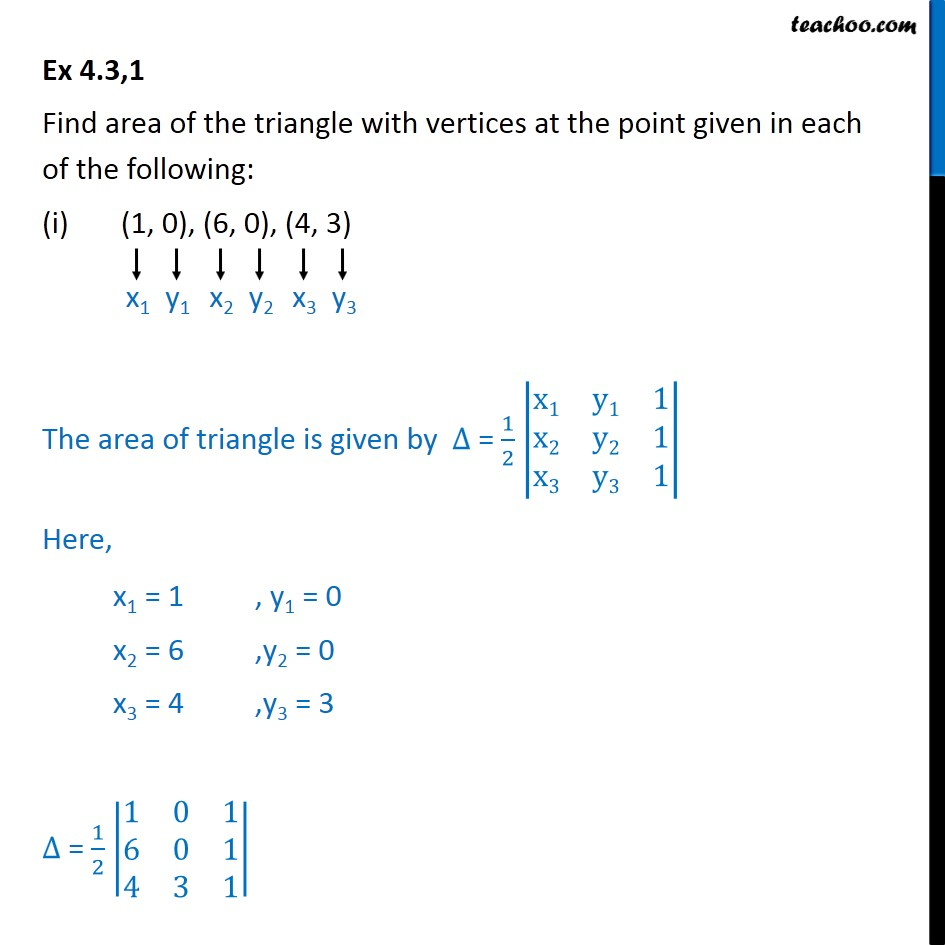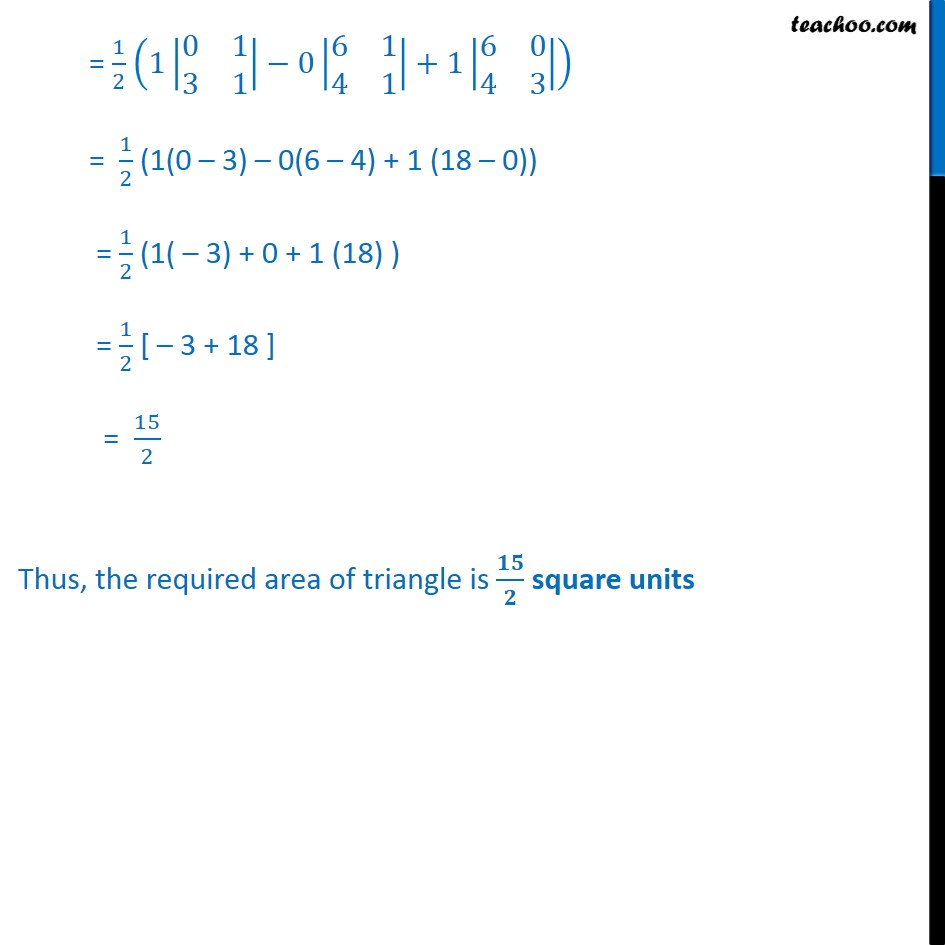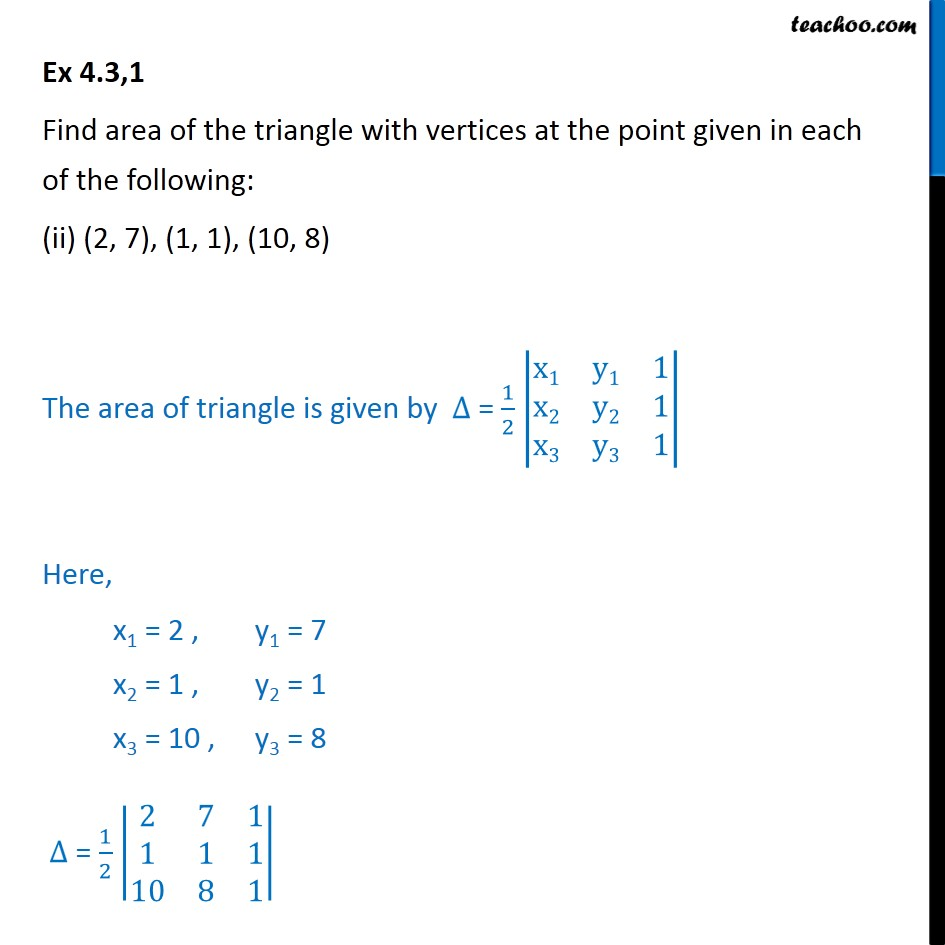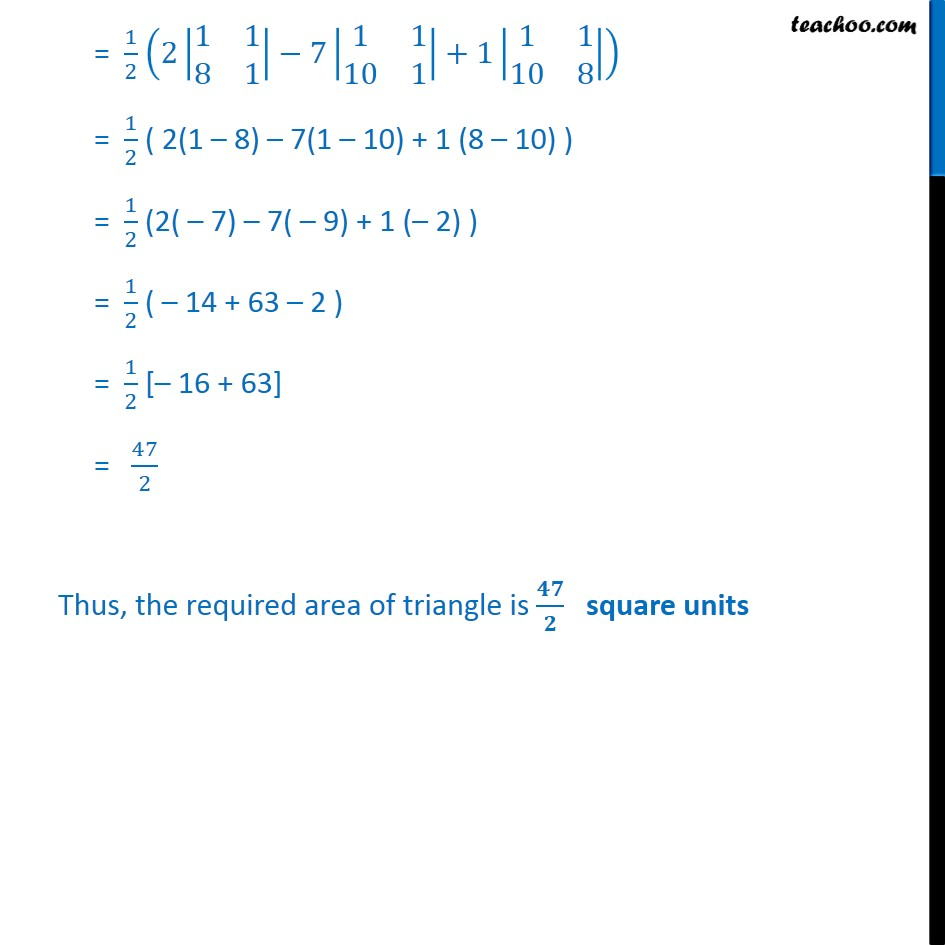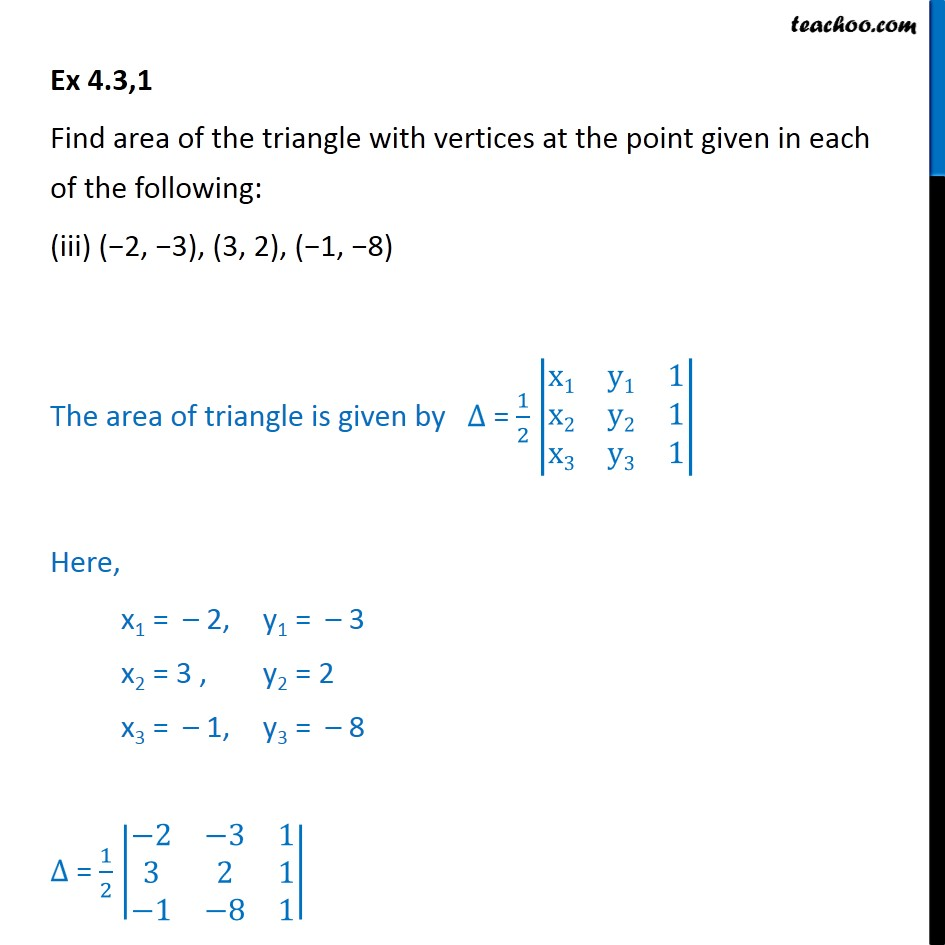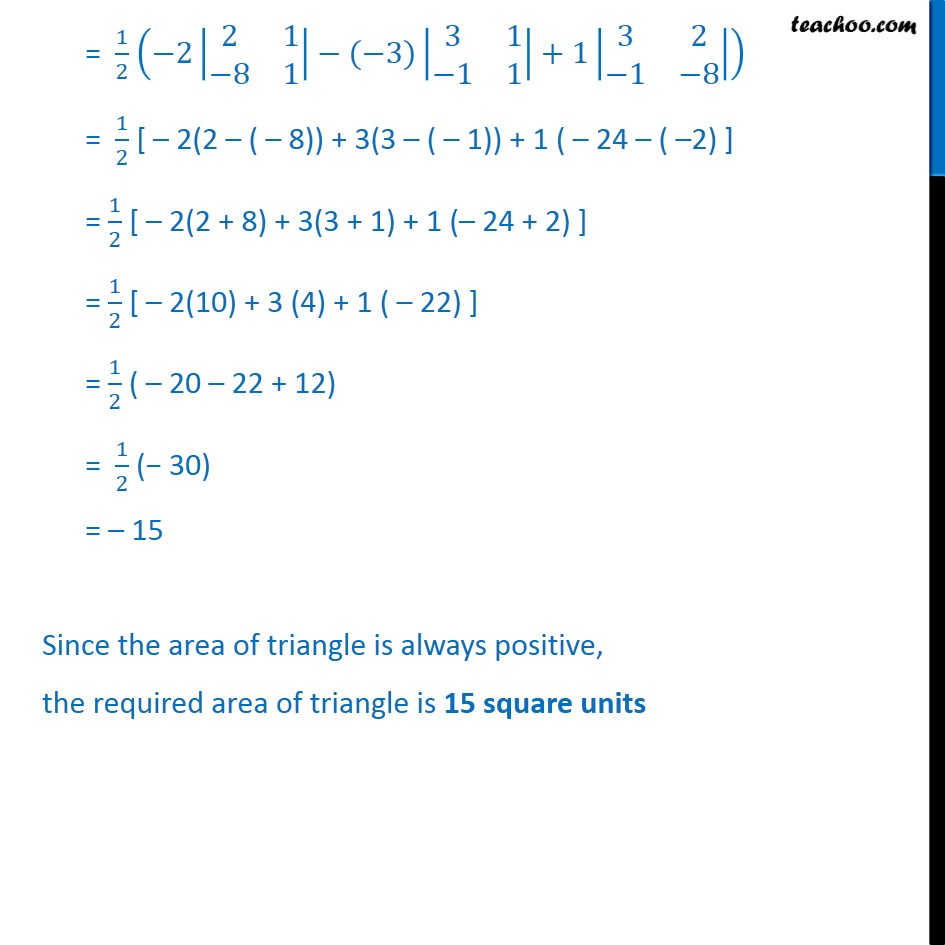Subscribe to our Youtube Channel - https://you.tube/teachoo

1. Chapter 4 Class 12 Determinants
2. Serial order wise
3. Ex 4.3

Transcript

Ex 4.3,1 Find area of the triangle with vertices at the point given in each of the following: • (1, 0), (6, 0), (4, 3) The area of triangle is given by ∆ = 1﷮2﷯ x1﷮y1﷮1﷮x2﷮y2﷮1﷮x3﷮y3﷮1﷯﷯ Here, x1 = 1 , y1 = 0 x2 = 6 ,y2 = 0 x3 = 4 ,y3 = 3 ∆ = 1﷮2﷯ 1﷮0﷮1﷮6﷮0﷮1﷮4﷮3﷮1﷯﷯ = 1﷮2﷯ 1 0﷮1﷮3﷮1﷯﷯−0 6﷮1﷮4﷮1﷯﷯+1 6﷮0﷮4﷮3﷯﷯﷯ = 1﷮2﷯ (1(0 – 3) – 0(6 – 4) + 1 (18 – 0)) = 1﷮2﷯ (1( – 3) + 0 + 1 (18) ) = 1﷮2﷯ [ – 3 + 18 ] = 15﷮2﷯ Thus, the required area of triangle is 𝟏𝟓﷮𝟐﷯ square units Ex 4.3,1 Find area of the triangle with vertices at the point given in each of the following: (ii) (2, 7), (1, 1), (10, 8) The area of triangle is given by ∆ = 1﷮2﷯ x1﷮y1﷮1﷮x2﷮y2﷮1﷮x3﷮y3﷮1﷯﷯ Here, x1 = 2 , y1 = 7 x2 = 1 , y2 = 1 x3 = 10 , y3 = 8 ∆ = 1﷮2﷯ 2﷮7﷮1﷮1﷮1﷮1﷮10﷮8﷮1﷯﷯ = 1﷮2﷯ 2 1﷮1﷮8﷮1﷯﷯−7 1﷮1﷮10﷮1﷯﷯+1 1﷮1﷮10﷮8﷯﷯﷯ = 1﷮2﷯ ( 2(1 – 8) – 7(1 – 10) + 1 (8 – 10) ) = 1﷮2﷯ (2( – 7) – 7( – 9) + 1 (– 2) ) = 1﷮2﷯ ( – 14 + 63 – 2 ) = 1﷮2﷯ [– 16 + 63] = 47﷮2﷯ Thus, the required area of triangle is 𝟒𝟕﷮𝟐﷯ square units Ex 4.3,1 Find area of the triangle with vertices at the point given in each of the following: (iii) (−2, −3), (3, 2), (−1, −8) The area of triangle is given by ∆ = 1﷮2﷯ x1﷮y1﷮1﷮x2﷮y2﷮1﷮x3﷮y3﷮1﷯﷯ Here, x1 = – 2, y1 = – 3 x2 = 3 , y2 = 2 x3 = – 1, y3 = – 8 ∆ = 1﷮2﷯ −2﷮−3﷮1﷮3﷮2﷮1﷮−1﷮−8﷮1﷯﷯ = 1﷮2﷯ −2 2﷮1﷮−8﷮1﷯﷯−(−3) 3﷮1﷮−1﷮1﷯﷯+1 3﷮2﷮−1﷮−8﷯﷯﷯ = 1﷮2﷯ [ – 2(2 – ( – 8)) + 3(3 – ( – 1)) + 1 ( – 24 – ( –2) ] = 1﷮2﷯ [ – 2(2 + 8) + 3(3 + 1) + 1 (– 24 + 2) ] = 1﷮2﷯ [ – 2(10) + 3 (4) + 1 ( – 22) ] = 1﷮2﷯ ( – 20 – 22 + 12) = 1﷮2﷯ (− 30) = – 15 Since the area of triangle is always positive, the required area of triangle is 15 square units

Ex 4.3